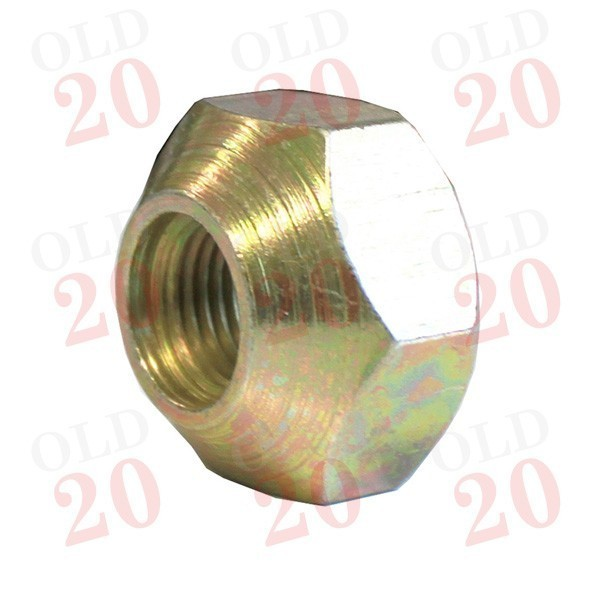View larger

# Wheel Nut

2086207

New

1/2" UNF

£ 1.20Tractors < Ferguson < TE20
Tractors < Ferguson < TEA20
Tractors < Ferguson < TED20
Tractors < Ferguson < TEF20
Tractors < Ferguson < FE35 (87mm)
Tractors < Ferguson < FE35 (23c)
Tractors < Massey Ferguson < Pre-100 Series < 35
Tractors < Massey Ferguson < Pre-100 Series < 35X
Tractors < Massey Ferguson < 100 < 135
Tractors < Massey Ferguson < 100 < 148
Tractors < Massey Ferguson < 100 < 165
Tractors < Massey Ferguson < 100 < 175
Tractors < Massey Ferguson < 100 < 178
Tractors < Massey Ferguson < 100 < 185
Tractors < Massey Ferguson < 100 < 188
Tractors < Massey Ferguson < 200 < 265
Tractors < Massey Ferguson < 200 < 275
Tractors < Massey Ferguson < 200 < 285
Tractors < Massey Ferguson < 200 < 290
Tractors < Massey Ferguson < 300 < 340
Tractors < Massey Ferguson < 300 < 342
Tractors < Massey Ferguson < 300 < 350
Tractors < Massey Ferguson < 300 < 352
Tractors < Massey Ferguson < 300 < 355
Tractors < Massey Ferguson < 300 < 360
Tractors < Massey Ferguson < 300 < 362
Tractors < Massey Ferguson < 300 < 365
Tractors < Massey Ferguson < 300 < 372N
Tractors < Massey Ferguson < 300 < 375
Tractors < Massey Ferguson < 300 < 390
Tractors < Massey Ferguson < 300 < 390T
Tractors < Massey Ferguson < 300 < 398
Tractors < Massey Ferguson < 300 < 399
Tractors < Massey Ferguson < 500 < 565
Tractors < Massey Ferguson < 500 < 575
Tractors < Massey Ferguson < 500 < 590
Tractors < Massey Ferguson < 600 < 675
Tractors < Massey Ferguson < 600 < 690

Supplier Reference :

 Section Front Axle & Steering
To suit Front Wheels